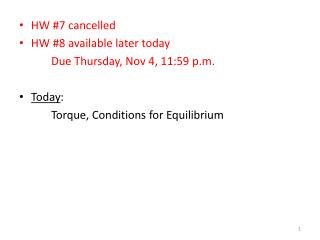DownloadDownload PresentationHW #7 cancelled HW #8 available later today Due Thursday, Nov 4, 11:59 p.m. Today :

# HW #7 cancelled HW #8 available later today Due Thursday, Nov 4, 11:59 p.m. Today :

Download Presentation## HW #7 cancelled HW #8 available later today Due Thursday, Nov 4, 11:59 p.m. Today :

- - - - - - - - - - - - - - - - - - - - - - - - - - - E N D - - - - - - - - - - - - - - - - - - - - - - - - - - -
##### Presentation Transcript

1. HW #7 cancelled • HW #8 available later today Due Thursday, Nov 4, 11:59 p.m. • Today: Torque, Conditions for Equilibrium

2. Forces and Torques In linear motion, a force causes acceleration. In rotational motion, a force applied some distance from an axis of rotation creates a torque, which causes rotational motion.

3. Torques Q: What happens ? F A: The see-saw rotates (CW direction). Q: To get the see-saw to rotate, would you want to apply the same force close to, or far from, the pivot ? F A: You would want to apply the force far from the pivot.

4. Definition of Torque F r O Let F be a force acting on an object, and let r be a position vector from a chosen point O to the point of application of the force, with F perpendicular to r . The magnitude of the torque τ exerted by the force F is r : length of the position vector F : magnitude of the force [ SI unit: Newton-meter (N-m) ]

5. Comments on Torque F2 F1 r2 r1 O 1 The vectors r and F lie in a plane. In a little bit, we will see that τ is perpendicular to this plane. The point O is usually chosen to coincide with the object’s axis of rotation (such as the pivot point above). 2 The object can rotate about O in either the CW or CCW directions. Convention : 3 CCW: τ > 0 CW: τ < 0

6. Analog to Linear Motion F1 r1 O If there are no torques, the rotational state (or angular speed) of the object does not change. Just like in linear motion, if there is no force, there is no change in the object’s motion (no acceleration, or no change in the velocity).

7. Torque: More Generally F1 r1 O What if the force F is not perpendicular to the position vector r ? The magnitude of the torque τ is then given by : θ : angle between F and r r1 Sign convention still applies : θ CCW: τ > 0 CW: τ < 0 F1

8. Direction of the Torque Technically, the torque τ is a vector, so it has a magnitude and direction. The direction is given by the RIGHT-HAND RULE. Point the fingers of your RIGHT HAND in the direction of r . Curl your fingers toward the direction of F . Your thumb then points in the direction of τ . F r OUT IN θ θ r F

9. Example Calculate the net torque (magnitude and direction) on this object: (a) For an axis of rotation through O1 perpendicular to the slide (b) For an axis of rotation through O2 perpendicular to the slide 40 N 45° O2 60° 30° O1 5 m 20 N 30 N 10 m

10. Forces, Torques, and Equilibrium “Balanced Rock” Arches National Park (Utah) 128 feet high “size of three school buses” An object in mechanical equilibrium must satisfy two conditions : The net external force must be zero : The net external torque must be zero : Σ : “sum of”

11. Example 40 kg 50 kg d = ? 2.0 m 10 kg (a) Compute the torque exerted on the plank by the red mass. (b) Where must the green mass be located, so that the plank does not rotate (i.e., in equilibrium) ? (c) Now assume the green mass is positioned so that the plank does not rotate. What is the normal force exerted by the pivot on the plank (i.e., in equilibrium) ?

12. Example: 8.6 Write the equations describing equilibrium for this object. Take the origin for the torque equation to be an axis perpendicular to this slide (or your paper) through the point O.

13. Conceptual: How is this safe ? California Science Center Los Angeles, CA

14. Conceptual: How is this safe ? Basic idea … What if bike is tilted to one side or the other ? CW τ CCW τ my mass m mg mg wheel wire = pivot wire = pivot wire counter-mass M Mg CW τ Mg CCW τ M > m |CCW τ| > |CW τ| |CW τ| > |CCW τ| Assume masses same distance from wire Important for M > m !!

15. Next Class • 8.3 – 8.4 : Center of Gravity, Equilibrium